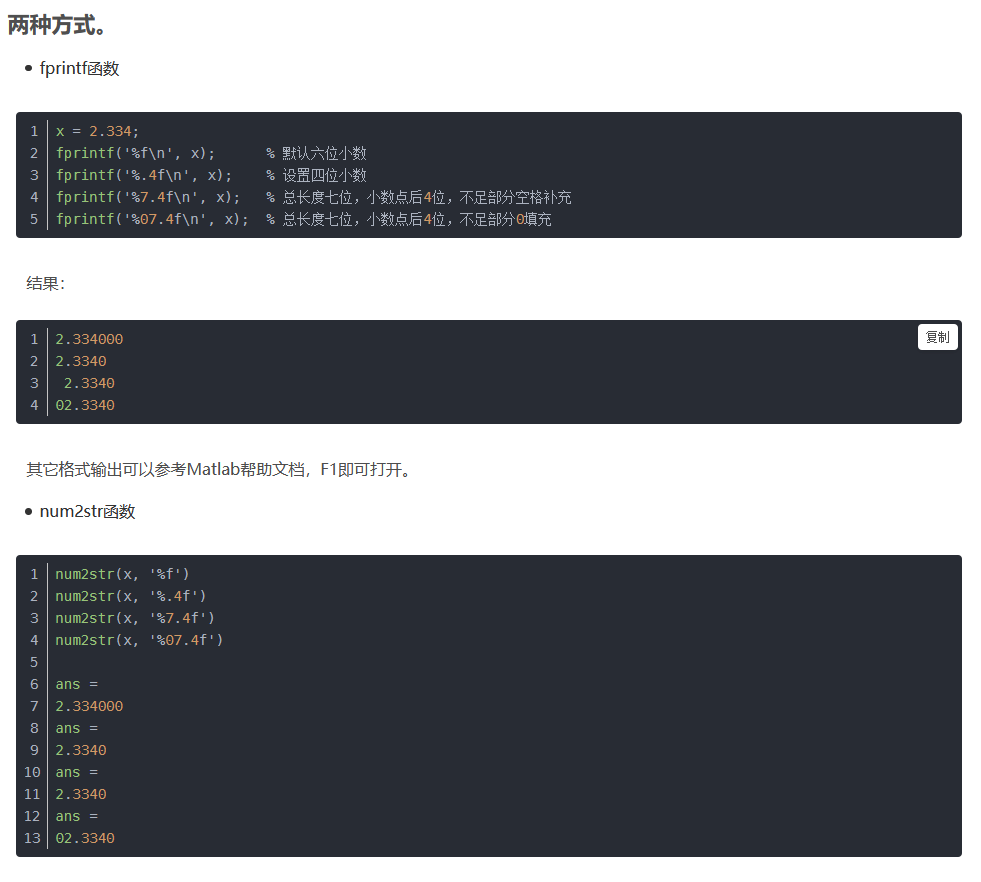• 使用matlab批量删除txt文件中连续的空格，只保留其中一个。如有两个连续的空格，删掉一个，留下一个。
• matlabtxt文件导入成mat文件，并且是double形式的。 1.需要先将txt文件存在m文件的文件夹里（工作路径）， ...3.将m文件里的txt文件删除，并将代码区修改。 load(‘D:\MATLAB\txtmat文件\Data1.mat’); ...
matlab将txt文件导入成mat文件，并且是double形式的。
1.需要先将txt文件存在m文件的文件夹里（工作路径），
load(‘t.txt’);
2.将已经在工作区的mat文件保存到固定的文件夹。
save(‘D:\MATLAB\txtmat文件\t.mat’)；
3.将m文件里的txt文件删除，并将代码区修改。
load(‘D:\MATLAB\txtmat文件\Data1.mat’);


展开全文• 使用matlab寻找序列中缺失的txt文件，同时批量删除txt文件中的空格或斜杠
• 文章参考:... 笔记留作个人科研使用,如有转载不允许,请告知,立刻删除. 文件读取方法 目标：读取每一行数字数目不同的纯文本文件。形如： 9 289734689 002874...
文章参考:https://blog.csdn.net/richard_yang2016/article/details/51763730

笔记留作个人科研使用,如有转载不允许,请告知,立刻删除.

文件读取方法

目标：读取每一行数字数目不同的纯文本文件。形如：

9

2   8  9   7   3  4   6   8   9

0   0  2   8   7  4   0   1   6

0   0  8   0   2  7   4   4   6

2   8  0   2   7  8   0   2   6

8   0  2   0   5  0   8   8   6

7   2  7   5   0  5   4   7   6

4   7  8   0   5  0   8   2   6

0   4  0   8   4  8   0   4   6

1   4  2   8   7  2   4   0   6

6   6  6   6   6  6   6   6   0

高级函数：
调用低级函数，语法简单，使用方便。

1. File-Import data

只适合纯数字（列数相同）或者开头只有几行文字（数字列数相同）的文件输入，保存为同文件名的.mat文件。

2. 函数 importdata

A=importdata(filename)

importdata('examp6_2_4.txt')

注意filename要加上.txt.

3. 函数load

load F_S9.txt

load -ascii  F_S9.txt  %强制以文本方式读取数据

注意：不能读取隔行数据不等长的情况

4. dlmread函数

M = dlmread (filename)

M = dlmread (filename, delimiter)

M = dlmread (filename, delimiter,R, C)  %R，C：指定读取开始点所在的行或者列, R=0 C=0表示第一行第一列。

M = dlmread (filename, delimiter, range)   %range=[R1,C1,R2,C2]

x = dlmread('examp6_2_3.txt')

x = dlmread('examp6_2_3.txt', ',', 2, 3)

x = dlmread('examp6_2_3.txt', ',', [1, 2, 2, 5])

数据不等长时，会自动以0补齐。

利用指定读取范围进行读取。

n=dlmread('singlerow_S9.txt','',[0,0,0,0])%读取第一个数字

l=dlmread('singlerow_S9.txt','',[2,0,2,n-1])%读取第三行数字

f=dlmread('singlerow_S9.txt','',4,0)  %读取第五行以后的数字

低级函数
语法复杂，按照各种格式读取文件，具有很好的灵活性。

1. 调用fopen 函数打开文件

fid = fopen ( filename, permission)   %fid 为正数，则找到这个文件。没有找到则返回-1.

[fid, message] = fopen('xiezhh.txt')

filename=['E:\software study\matlab\','6_2_1','.txt'];

disp (filename)；

fid=fopen(filename)；

2. 调用fclose 关闭打开的文件。

Close（fid）关闭文件标识符fid指定的文件。

Close(all) 关闭所有被打开的文件

filename=['E:\software study\matlab\','6_2_1','.txt'];

disp (filename)

fid=fopen(filename)

fclose(fid)

3. 调用fgets 和fgetl 函数读取文件的下一行

Fgets 读取下一行，包括换行符，fgetl 不包括换行符。

Tline=fgetl(fid)

Tline=fgetl(fid,nchar)  %nchar 表示只读取前nchar个字符，其他的会丢掉。

filename=['E:\software study\matlab\','6_2_1','.txt'];

disp (filename);

fid=fopen(filename);

n=fgets(fid)

fgetl(fid)

l=fgetl(fid)

fclose(fid);

不能对几行的范围同时操作。只能得到第一和第三行的信息。

filename=['E:\software study\matlab\Learning code\File_Exchange\','singlerow_S9','.txt'];

disp (filename);

fid=fopen(filename);

n=fgetl(fid)

fgetl(fid);

l=fgetl(fid)

fclose(fid);


展开全文• MATLAB判断文件是否存在： exist('output.txt','file') 如果output.txt存在，返回2，若不存在返回0 MATLAB删除文件： delete('output.txt');
MATLAB判断文件是否存在：

exist('output.txt','file')

如果output.txt存在，返回2，若不存在返回0

MATLAB删除文件：

delete('output.txt');


展开全文• 因为用matlab比较多，之前也用matlab处理过txt文件（读取，然后在matlab里处理，不需要输出）， 我就在想我先将数据读取到matlab，然后将自己需要的列组成了矩阵，输出到txt中。 1.读取 [time,CO2, PM25, PM10, ...
昨天吃饭接到数据处理任务，从txt中删除一些列，需求简单，但对于我一个代码小白来说，很难。

因为用matlab比较多，之前也用matlab处理过txt文件（读取，然后在matlab里处理，不需要输出），

我就在想我先将数据读取到matlab，然后将自己需要的列组成了矩阵，输出到txt中。

1.读取

[time,CO2, PM25, PM10, H2S,SO2, NO2, O3, CO, VOC,Tep,Humidity,Airp,lat,lon,height] = textread('园区.txt','%f %f %f %f... %f %f %f %f %f %f %f %f %f %f %f %f','headerlines',1);

用txtread读取，'headerlines',1代表跳过第一行（标题行）读取数据

2.新矩阵

y=[time,PM25,PM10,SO2,VOC,Tep,Humidity,Airp,lat,lon,height];

组成一个矩阵

3.输出

[m,n]=size(y);
for i=1:1:m
fprintf(fid,'%d %d %d %d %.3f %05.1f %05.1f %d %f %f %.3f\n',y(i,:));
end
fclose(fid);

每行输出，然后可以设置每列的数据精度。

附

每个元素输入：

fid=fopen('D:\新建文件夹\数据\0.txt','w');%写入文件路径
a=int8(dd);
[m,n]=size(a);
for i=1:1:m
for j=1:1:n
if j==n
fprintf(fid,'%g\r\n',a(i,j));
else
fprintf(fid,'%g',a(i,j));
end
end
end
fclose(fid);

参考：MATLAB-txt读取,输出txt（以矩阵形式）

关于数据精度

参考：Matlab学习手记——特定格式输出

这里要注意一点：小数点也要算一位展开全文• close all clear all clc A=[]; b=1; for i=1:499  m = num2str(i,'%06d');  fid = fopen(['G:\实验数据\txt\',m,'.txt'],'r');  if fid  A(b)=i;  b=b+1;  end end % fid=fopen
• % 生成文件（用于测试） fp = fopen('test.txt','w'); fclose(fp); % 新建文件夹（路径） subPath1 = fullfile(rootPath,'1'); subPath2 = fullfile(rootPath,'2'); if ~exist(subPath1,'dir') mkdir(subPath1) %...
• 写在前面的话：文章内容来源于但不限于网络、书籍、个人心得体会等，意在总结和方便各位同行快速参考...VOC格式数据集，相关TXT文件生成Matlab脚本。 xmlfilepath='F:\VOCdevkit\VOC2007\Annotations'; txtsavepa...
• matlab修改多级文件夹路径下的文件名： % %%%%%%%%%%%%%%批量修改文件名一级文件夹 \路径下直接为文件 % close all;clear all;clc; % path='G:\100万采样\YC_AK采样\value-100\version-15\direction-1\新建...
• 一、matlab导入并处理xml文件数据xmlDoc = xmlread('position_sen2_mod1.xml'); %position_sen2_mod1.xml为删除了network.dtd这一行的文件NodeArray= xmlDoc.getElementsByTagName('node'); %将所有node节点放入数组...
• 1.读文件temp1 = load('G:\seu\高频涂覆材料\修改3\angle.txt');2.写文件fp = fopen('G:\seu\高频涂覆材料\修改3\s_para...3.删除文件delete('G:\seu\高频涂覆材料\修改2\aa.txt');4.重命名文件movefile('G:\seu\高频涂
• 批量删除matlab注释：（注释符是%） 1.把代码拷到visual studio的一个txt文件里。（用vs code，或sublime也可以代替visual studio） 2.用ctrl+H可以调出文本替换的对话框 3.选择搜索的模式：正则表达式 4. 搜索框...
• 去除数组某个元素： &gt;&gt;a = [1:10] %生成数组a a = 1 2 3 4 5 6 7 8 9 10 &gt;... %删除数组a中第5个元素 ...读取文件txt里面的内容是： 6 book 4 water 3 food 7 computer 9 snack...
• 删除文件加中所有.jpg 后缀的图片。如遇需要，更改后缀为 .mat/.png/.txt 等等。delete(‘E:\matlabCode_2016\images*.jpg’)；
• 通过传统的Matlab GUI ，设计了一个小工具，主要用于对txt文本和m脚本执行修改操作 当前版本功能要点如下： 1：支持精确匹配和模糊匹配的方式，查找并替换字符串； 2：支持精确匹配和模糊匹配的方式，删除匹配字...python linux java mysql 数据库
•  (1) 从文本文件input.txt读入。第一行包含一个数 N，表示商品个数。之后跟着 N 行，每行包含一个字符串（商品名称）和一个数（商品价格）。  (2) 删除价格是3的倍数的商品；  (3) 显示剩余商品的个数 K；  ...
• matlab例子，把变量存入到一系列有规律文件名的文件matlab 如何以动态的变量名保存变量matlab如何删除变量matlab中字符串用单引号使用 今天通过天使一个程序，学习了许多关于matlab的使用的技巧，记录一下，希望...
• MATLAB处理串口采集数据 加速度计反馈X/Y/Z轴数据，并通过串口输出，...第一步：将采集到的的串口数据保存成.txt文件，需要删除txt文件中开头不完整数据，确保第一组数据为标准数据格式。 完整数据以CC开头，共11个字数据分析
• 1.修改TXT数据 删除TXT文档中选中的不规律段不规律段有两段分别位于 文档首尾然后保存文件 2.将TXT数据导入MATLABMatlab命令窗口输入 data1 load'f:\sheng\PDDM\2013021113\number1.txt; 引号内的内容为TXT文档...
• every blog every motto: The shortest answer...原始点数据新建一个txt文件，保存后删除后缀，放在./data文件夹下 迭代1次，效果 迭代三次效果 1.1.2 代码部分 % This is a fline.m program (fractal line interpo
• ## 聚类分析Matlab代码

千次阅读 2019-08-19 20:55:49
load demo2.txt %把原始数据保存在纯文本文件demo2.txt中 demo2(:,[3:6])=[]; %删除数据矩阵的第3列～第6列,即使用变量1,2,7,8,9,10 demo2=zscore(demo2); %数据标准化 y=pdist(demo2); %求对象间...聚类分析 数学建模
• txt文件的写入，打开，删除操作 1.建立一张图像的X,Y坐标矩阵 假如a是一个两行四列的矩阵，则 2.在特定路径下找到特定后缀名的文件名及数量 function [names,class_num] = GetFiles_path(path) files = dir([path...
• 而且只有关掉日志，才能删除日志文件。 4,查看当前日志是开或者关闭的状态：get(0,‘Diary’) 由于我在此之前已经关闭了日志，所以状态显示关闭。 5，查看当前 diary 文件的名称： get(0,‘DiaryFile’) ...
• 高级文件I/O介绍了读写MAT、ASCII、TXT、Excel、图片和音频等文件的方法及相关函数；低级文件I/O介绍了读写二进制和文本文件的方法及相关函数。之后以专题形式，全面讲解了读写文本文件的技巧与方法。 第3章：二维...
• 一-得到一个矩阵 ...3-写在一个txt里面，数组名就是文件名，然后clear之后可以load some.txt 二-删除 调用格式：A(:, n) = [], A(m, :) = []  A(:, n) = []表示删除矩阵A的第n列  A(m, :) = []表示删除矩阵...
• 比如我们一组数据，里面有不少的NaN值，如何将其删除掉呢？可以通过find函数来搞定。 我们可以通过importdata('data.txt')将数据文件data.txt导入数组A中。 A=importdata('data.txt') 然后通过命令 z=find(~isna...
• 比如我们一组数据，里面有不少的NaN值，如何将其删除掉呢？... 我们可以通过importdata('data.txt')将数据文件data.txt导入数组A中。  A=importdata('data.txt')  然后通过命令  z=find(~isnan...
• 不停服务清空nohup产生的日志...会生成一个日志文件log.txt,后来想删除而不停止服务，运行如下代码解决： cp /dev/null log.txt （前提是cd 进log.txt所在目录文件夹下） 第二种方法： cat /dev/null &g...
• 对象的matlab跑数据会出来上百个txt文件，前面都是固定格式的13行无用，需要删掉，尝试着用autohotkeky实现了一下 代码如下 loop,%a_workingdir%\*.txt, ;遍历当前脚本工作文件夹的文本文档 { a=0 ;当前计数器置...autohotkey# matlabtxt删除文件matlab 订阅